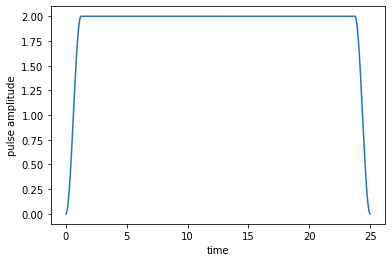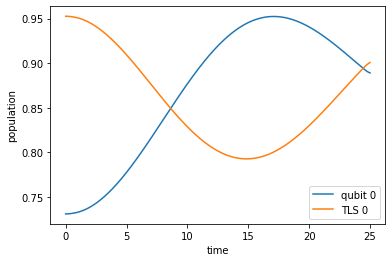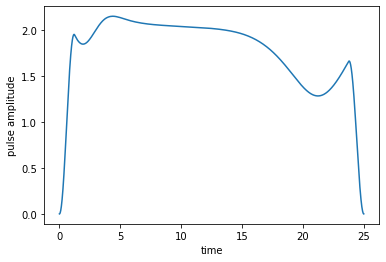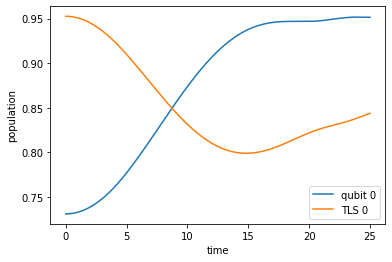# Optimization of Dissipative Qubit Reset¶

In :
# NBVAL_IGNORE_OUTPUT
import qutip
import numpy as np
import scipy
import matplotlib
import matplotlib.pylab as plt
import krotov

%watermark -v --iversions

matplotlib       3.3.1
qutip            4.5.0
matplotlib.pylab 1.17.2
scipy            1.3.1
numpy            1.17.2
krotov           1.2.0
CPython 3.7.6
IPython 7.17.0


$\newcommand{tr}{\operatorname{tr}} \newcommand{diag}{\operatorname{diag}} \newcommand{abs}{\operatorname{abs}} \newcommand{pop}{\operatorname{pop}} \newcommand{aux}{\text{aux}} \newcommand{int}{\text{int}} \newcommand{opt}{\text{opt}} \newcommand{tgt}{\text{tgt}} \newcommand{init}{\text{init}} \newcommand{lab}{\text{lab}} \newcommand{rwa}{\text{rwa}} \newcommand{bra}{\langle#1\vert} \newcommand{ket}{\vert#1\rangle} \newcommand{Bra}{\left\langle#1\right\vert} \newcommand{Ket}{\left\vert#1\right\rangle} \newcommand{Braket}{\left\langle #1\vphantom{#2} \mid # 2\vphantom{#1}\right\rangle}¶ \newcommand{op}{\hat{#1}} \newcommand{Op}{\hat{#1}} \newcommand{dd}{\,\text{d}} \newcommand{Liouville}{\mathcal{L}} \newcommand{DynMap}{\mathcal{E}} \newcommand{identity}{\mathbf{1}} \newcommand{Norm}{\lVert#1\rVert} \newcommand{Abs}{\left\vert#1\right\vert} \newcommand{avg}{\langle#1\rangle} \newcommand{Avg}{\left\langle#1\right\rangle} \newcommand{AbsSq}{\left\vert#1\right\vert^2} \newcommand{Re}{\operatorname{Re}} \newcommand{Im}{\operatorname{Im}}$

This example illustrates an optimization in an open quantum system, where the dynamics is governed by the Liouville-von Neumann equation. Hence, states are represented by density matrices $\op{\rho}(t)$ and the time-evolution operator is given by a general dynamical map $\DynMap$.

## Define parameters¶

The system consists of a qubit with Hamiltonian $\op{H}_{q}(t) = - \frac{\omega_{q}}{2} \op{\sigma}_{z} - \frac{\epsilon(t)}{2} \op{\sigma}_{z}$, where $\omega_{q}$ is an energy level splitting that can be dynamically adjusted by the control $\epsilon(t)$. This qubit couples strongly to another two-level system (TLS) with Hamiltonian $\op{H}_{t} = - \frac{\omega_{t}}{2} \op{\sigma}_{z}$ with static energy level splitting $\omega_{t}$. The coupling strength between both systems is given by $J$ with the interaction Hamiltonian given by $\op{H}_{\int} = J \op{\sigma}_{x} \otimes \op{\sigma}_{x}$.

The Hamiltonian for the system of qubit and TLS is

$$\op{H}(t) = \op{H}_{q}(t) \otimes \identity_{t} + \identity_{q} \otimes \op{H}_{t} + \op{H}_{\int}.$$

In addition, the TLS is embedded in a heat bath with inverse temperature $\beta$. The TLS couples to the bath with rate $\kappa$. In order to simulate the dissipation arising from this coupling, we consider the two Lindblad operators

$$\begin{split} \op{L}_{1} &= \sqrt{\kappa (N_{th}+1)} \identity_{q} \otimes \ket{0}\bra{1} \\ \op{L}_{2} &= \sqrt{\kappa N_{th}} \identity_{q} \otimes \ket{1}\bra{0} \end{split}$$

with $N_{th} = 1/(e^{\beta \omega_{t}} - 1)$.

In :
omega_q = 1.0  # qubit level splitting
omega_T = 3.0  # TLS level splitting
J = 0.1  # qubit-TLS coupling
kappa = 0.04  # TLS decay rate
beta = 1.0  # inverse bath temperature
T = 25.0  # final time
nt = 2500  # number of time steps


## Define the Liouvillian¶

The dynamics of the qubit-TLS system state $\op{\rho}(t)$ is governed by the Liouville-von Neumann equation

$$\begin{split} \frac{\partial}{\partial t} \op{\rho}(t) &= \Liouville(t) \op{\rho}(t) \\ &= - i \left[\op{H}(t), \op{\rho}(t)\right] + \sum_{k=1,2} \left( \op{L}_{k} \op{\rho}(t) \op{L}_{k}^\dagger - \frac{1}{2} \op{L}_{k}^\dagger \op{L}_{k} \op{\rho}(t) - \frac{1}{2} \op{\rho}(t) \op{L}_{k}^\dagger \op{L}_{k} \right)\,. \end{split}$$
In :
def liouvillian(omega_q, omega_T, J, kappa, beta):
"""Liouvillian for the coupled system of qubit and TLS"""

# drift qubit Hamiltonian
H0_q = 0.5 * omega_q * np.diag([-1, 1])
# drive qubit Hamiltonian
H1_q = 0.5 * np.diag([-1, 1])

# drift TLS Hamiltonian
H0_T = 0.5 * omega_T * np.diag([-1, 1])

# Lift Hamiltonians to joint system operators
H0 = np.kron(H0_q, np.identity(2)) + np.kron(np.identity(2), H0_T)
H1 = np.kron(H1_q, np.identity(2))

# qubit-TLS interaction
H_int = J * np.fliplr(np.diag([0, 1, 1, 0]))

# convert Hamiltonians to QuTiP objects
H0 = qutip.Qobj(H0 + H_int)
H1 = qutip.Qobj(H1)

N = 1.0 / (np.exp(beta * omega_T) - 1.0)
# Cooling on TLS
L1 = np.sqrt(kappa * (N + 1)) * np.kron(
np.identity(2), np.array([[0, 1], [0, 0]])
)
# Heating on TLS
L2 = np.sqrt(kappa * N) * np.kron(
np.identity(2), np.array([[0, 0], [1, 0]])
)

# convert Lindblad operators to QuTiP objects
L1 = qutip.Qobj(L1)
L2 = qutip.Qobj(L2)

# generate the Liouvillian
L0 = qutip.liouvillian(H=H0, c_ops=[L1, L2])
L1 = qutip.liouvillian(H=H1)

# Shift the qubit and TLS into resonance by default
eps0 = lambda t, args: omega_T - omega_q

return [L0, [L1, eps0]]

L = liouvillian(omega_q=omega_q, omega_T=omega_T, J=J, kappa=kappa, beta=beta)


## Define the optimization target¶

The initial state of qubit and TLS are assumed to be in thermal equilibrium with the heat bath (although only the TLS is directly interacting with the bath). Both states are given by

$$\op{\rho}_{\alpha}^{th} = \frac{e^{x_{\alpha}} \ket{0}\bra{0} + e^{-x_{\alpha}} \ket{1}\bra{1}}{2 \cosh(x_{\alpha})}, \qquad x_{\alpha} = \frac{\omega_{\alpha} \beta}{2},$$

with $\alpha = q,t$. The initial state of the bipartite system of qubit and TLS is given by the thermal state $\op{\rho}_{th} = \op{\rho}_{q}^{th} \otimes \op{\rho}_{t}^{th}$.

In :
x_q = omega_q * beta / 2.0
rho_q_th = np.diag([np.exp(x_q), np.exp(-x_q)]) / (2 * np.cosh(x_q))

x_T = omega_T * beta / 2.0
rho_T_th = np.diag([np.exp(x_T), np.exp(-x_T)]) / (2 * np.cosh(x_T))

rho_th = qutip.Qobj(np.kron(rho_q_th, rho_T_th))


Since we are ultimately only interested in the state of the qubit, we define trace_TLS. It returns the reduced state of the qubit $\op{\rho}_{q} = \tr_{t}\{\op{\rho}\}$ when passed the state $\op{\rho}$ of the bipartite system.

In :
def trace_TLS(rho):
"""Partial trace over the TLS degrees of freedom"""
rho_q = np.zeros(shape=(2, 2), dtype=np.complex_)
rho_q[0, 0] = rho[0, 0] + rho[1, 1]
rho_q[0, 1] = rho[0, 2] + rho[1, 3]
rho_q[1, 0] = rho[2, 0] + rho[3, 1]
rho_q[1, 1] = rho[2, 2] + rho[3, 3]
return qutip.Qobj(rho_q)


The target state is (temporarily) the ground state of the bipartite system, i.e., $\op{\rho}_{\tgt} = \ket{00}\bra{00}$. Note that in the end we will only optimize the reduced state of the qubit.

In :
rho_q_trg = np.diag([1, 0])
rho_T_trg = np.diag([1, 0])
rho_trg = np.kron(rho_q_trg, rho_T_trg)
rho_trg = qutip.Qobj(rho_trg)


Next, the list of objectives is defined, which contains the initial and target state and the Liouvillian $\Liouville(t)$ that determines the system dynamics.

In :
objectives = [krotov.Objective(initial_state=rho_th, target=rho_trg, H=L)]
objectives

Out:
[Objective[ρ₀[4,4] to ρ₁[4,4] via [𝓛₀[[4,4],[4,4]], [𝓛₁[[4,4],[4,4]], u₁(t)]]]]

In the following, we define the shape function $S(t)$, which we use in order to ensure a smooth switch on and off in the beginning and end. Note that at times $t$ where $S(t)$ vanishes, the updates of the field is suppressed.

In :
def S(t):
"""Shape function for the field update"""
return krotov.shapes.flattop(
t, t_start=0, t_stop=T, t_rise=0.05 * T, t_fall=0.05 * T, func='sinsq'
)


We re-use this function to also shape the guess control $\epsilon_{0}(t)$ to be zero at $t=0$ and $t=T$. This is on top of the originally defined constant value shifting the qubit and TLS into resonance.

In :
def shape_field(eps0):
"""Applies the shape function S(t) to the guess field"""
eps0_shaped = lambda t, args: eps0(t, args) * S(t)
return eps0_shaped

L = shape_field(L)


At last, before heading to the actual optimization below, we assign the shape function $S(t)$ to the OCT parameters of the control and choose lambda_a, a numerical parameter that controls the field update magnitude in each iteration.

In :
pulse_options = {L: dict(lambda_a=0.01, update_shape=S)}


## Simulate the dynamics of the guess field¶

In :
tlist = np.linspace(0, T, nt)

In :
def plot_pulse(pulse, tlist):
fig, ax = plt.subplots()
if callable(pulse):
pulse = np.array([pulse(t, args=None) for t in tlist])
ax.plot(tlist, pulse)
ax.set_xlabel('time')
ax.set_ylabel('pulse amplitude')
plt.show(fig)


The following plot shows the guess field $\epsilon_{0}(t)$ as a constant that puts qubit and TLS into resonance, but with a smooth switch-on and switch-off.

In :
plot_pulse(L, tlist)We solve the equation of motion for this guess field, storing the expectation values for the population in the bipartite levels:

In :
psi00 = qutip.Qobj(np.kron(np.array([1,0]), np.array([1,0])))
psi01 = qutip.Qobj(np.kron(np.array([1,0]), np.array([0,1])))
psi10 = qutip.Qobj(np.kron(np.array([0,1]), np.array([1,0])))
psi11 = qutip.Qobj(np.kron(np.array([0,1]), np.array([0,1])))
proj_00 = qutip.ket2dm(psi00)
proj_01 = qutip.ket2dm(psi01)
proj_10 = qutip.ket2dm(psi10)
proj_11 = qutip.ket2dm(psi11)

In :
guess_dynamics = objectives.mesolve(
tlist, e_ops=[proj_00, proj_01, proj_10, proj_11]
)

In :
def plot_population(result):
fig, ax = plt.subplots()
ax.plot(
result.times,
np.array(result.expect) + np.array(result.expect),
label='qubit 0',
)
ax.plot(
result.times,
np.array(result.expect) + np.array(result.expect),
label='TLS 0',
)
ax.legend()
ax.set_xlabel('time')
ax.set_ylabel('population')
plt.show(fig)

plot_population(guess_dynamics)The population dynamics of qubit and TLS ground state show that both are oscillating and especially the qubit's ground state population reaches a maximal value at intermediate times $t < T$. This maximum is indeed the maximum that is physically possible. It corresponds to a perfect swap of the initial qubit and TLS purities. However, we want to reach this maximum at final time $T$ (not before), so the guess control is not yet working as desired.

## Optimize¶

Our optimization target is the ground state $\ket{\Psi_{q}^{\tgt}} = \ket{0}$ of the qubit, irrespective of the state of the TLS. Thus, our optimization functional reads

$$F_{re} = 1 - \Braket{\Psi_{q}^{\tgt}}{\tr_{t}\{\op{\rho}(T)\} \,|\; \Psi_{q}^{\tgt}}\,,$$

and we first define print_qubit_error, which prints out the above functional after each iteration.

In :
def print_qubit_error(**args):
"""Utility function writing the qubit error to screen"""
taus = []
for state_T in args['fw_states_T']:
state_q_T = trace_TLS(state_T)
taus.append(state_q_T[0, 0].real)
J_T_re = 1 - np.average(taus)
print("    qubit error: %.1e" % J_T_re)
return J_T_re


In order to minimize the above functional, we need to provide the correct chi_constructor for the Krotov optimization. This is the only place where the functional (implicitly) enters the optimization. Given our bipartite system and choice of $F_{re}$, the equation for $\op{\chi}(T)$ reads

$$\op{\chi}(T) = \sum_{k=0,1} a_{k} \op{\rho}_{q}^{\tgt} \otimes \ket{k}\bra{k}$$

with $\{\ket{k}\}$ a basis for the TLS Hilbert space.

In :
def TLS_onb_trg():
"""Returns the tensor product of qubit target state
and a basis for the TLS Hilbert space"""
rho1 = qutip.Qobj(np.kron(rho_q_trg, np.diag([1, 0])))
rho2 = qutip.Qobj(np.kron(rho_q_trg, np.diag([0, 1])))
return [rho1, rho2]

TLS_onb = TLS_onb_trg()

def chis_qubit(fw_states_T, objectives, tau_vals):
"""Calculate chis for the chosen functional"""
chis = []
for state_i_T in fw_states_T:
chis_i = np.zeros(shape=(4, 4), dtype=np.complex_)
for state_k in TLS_onb:
a_i_k = krotov.optimize._overlap(state_i_T, state_k)
chis_i += a_i_k * state_k
chis.append(qutip.Qobj(chis_i))
return chis


We now carry out the optimization for five iterations.

In :
# NBVAL_IGNORE_OUTPUT
# the DensityMatrixODEPropagator is not sufficiently exact to guarantee that
# you won't get slightly different results in the optimization when
# running this on different systems
opt_result = krotov.optimize_pulses(
objectives,
pulse_options,
tlist,
propagator=krotov.propagators.DensityMatrixODEPropagator(
atol=1e-10, rtol=1e-8
),
chi_constructor=chis_qubit,
info_hook=krotov.info_hooks.chain(
krotov.info_hooks.print_debug_information, print_qubit_error
),
check_convergence=krotov.convergence.check_monotonic_error,
iter_stop=5,
)

Iteration 0
objectives:
1:ρ₀[4,4] to ρ₁[4,4] via [𝓛₀[[4,4],[4,4]], [𝓛₁[[4,4],[4,4]], u₂(t)]]
1:ρ₂[4,4] to ρ₃[4,4] via [𝓛₂[[4,4],[4,4]], [𝓛₃[[4,4],[4,4]], u₂(t)]]
chi_constructor: chis_qubit
mu: derivative_wrt_pulse
S(t) (ranges): [0.000000, 1.000000]
iter_start: 0
iter_stop: 5
duration: 0.3 secs (started at 2020-08-17 10:41:30)
optimized pulses (ranges): [0.00, 2.00]
∫gₐ(t)dt: 0.00e+00
λₐ: 1.00e-02
storage (bw, fw, fw0): None, None, None
fw_states_T norm: 1.000000
τ: (7.97e-01:0.00π)
qubit error: 1.1e-01
Iteration 1
duration: 2.5 secs (started at 2020-08-17 10:41:31)
optimized pulses (ranges): [0.00, 2.06]
∫gₐ(t)dt: 7.64e-02
λₐ: 1.00e-02
storage (bw, fw, fw0): [1 * ndarray(2500)] (1.3 MB), None, None
fw_states_T norm: 1.000000
τ: (7.98e-01:0.00π)
qubit error: 1.1e-01
Iteration 2
duration: 2.9 secs (started at 2020-08-17 10:41:33)
optimized pulses (ranges): [0.00, 2.23]
∫gₐ(t)dt: 5.71e-01
λₐ: 1.00e-02
storage (bw, fw, fw0): [1 * ndarray(2500)] (1.3 MB), None, None
fw_states_T norm: 1.000000
τ: (8.01e-01:0.00π)
qubit error: 6.7e-02
Iteration 3
duration: 2.9 secs (started at 2020-08-17 10:41:36)
optimized pulses (ranges): [0.00, 2.33]
∫gₐ(t)dt: 8.19e-02
λₐ: 1.00e-02
storage (bw, fw, fw0): [1 * ndarray(2500)] (1.3 MB), None, None
fw_states_T norm: 1.000000
τ: (7.99e-01:0.00π)
qubit error: 5.0e-02
Iteration 4
duration: 2.9 secs (started at 2020-08-17 10:41:39)
optimized pulses (ranges): [0.00, 2.19]
∫gₐ(t)dt: 2.16e-01
λₐ: 1.00e-02
storage (bw, fw, fw0): [1 * ndarray(2500)] (1.3 MB), None, None
fw_states_T norm: 1.000000
τ: (8.02e-01:0.00π)
qubit error: 4.9e-02
Iteration 5
duration: 3.0 secs (started at 2020-08-17 10:41:42)
optimized pulses (ranges): [0.00, 2.15]
∫gₐ(t)dt: 6.40e-02
λₐ: 1.00e-02
storage (bw, fw, fw0): [1 * ndarray(2500)] (1.3 MB), None, None
fw_states_T norm: 1.000000
τ: (8.03e-01:0.00π)
qubit error: 4.9e-02

In :
opt_result

Out:
Krotov Optimization Result
--------------------------
- Started at 2020-08-17 10:41:30
- Number of objectives: 1
- Number of iterations: 5
- Reason for termination: Reached 5 iterations
- Ended at 2020-08-17 10:41:45 (0:00:15)

## Simulate the dynamics of the optimized field¶

The plot of the optimized field shows that the optimization slightly shifts the field such that qubit and TLS are no longer perfectly in resonance.

In :
plot_pulse(opt_result.optimized_controls, tlist)This slight shift of qubit and TLS out of resonance delays the population oscillations between qubit and TLS ground state such that the qubit ground state is maximally populated at final time $T$.

In :
optimized_dynamics = opt_result.optimized_objectives.mesolve(
tlist, e_ops=[proj_00, proj_01, proj_10, proj_11]
)

plot_population(optimized_dynamics)